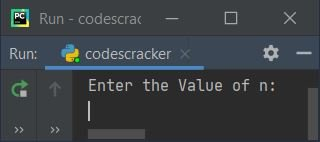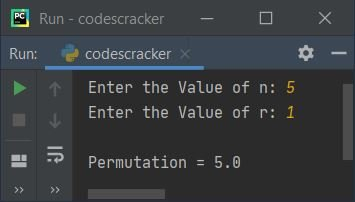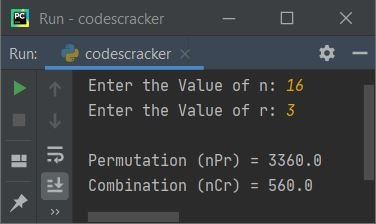# Python Program to Find nCr and nPr

This article is created to cover some programs in Python, that find and prints the value of nCr (Combination) and nPr (Permutation) based on the value of n and r entered by user at run-time. Here are the list of programs:

• Find nPr (Permutation)
• Find nCr (Combination)
• Find nPr and nCr using Function

Before creating these programs, let's remind about permutation and combination in brief.

### nPr and nCr Formula

The nPr (permutation) formula is:

`nPr = n!/(n-r)!`

The exclamation (!) symbol indicates factorial. For example, 5! can be called as 5 factorial, that equals 120 (5*4*3*2*1)

The nCr (combination) formula is:

`nCr = n!/r!(n-r)!`

Important - The nPr value shows the number of ways to arrange r things out of n

Important - The nCr value shows the number of ways to select r things out of n

## Find nPr (Permutation)

This program find and prints nPr value based on the value of n and r. The n and r value must be entered by user. The question is, write a Python program to find nPr. Here is its answer:

```print("Enter the Value of n: ")
n = int(input())
print("Enter the Value of r: ")
r = int(input())

fact = 1
i = 1
while i<=n:
fact = i*fact
i = i+1

numerator = fact          # n!
sub = n - r               # (n-r)
fact = 1
i = 1
while i<=sub:
fact = i*fact
i = i+1
denominator = fact        # (n-r)!
perm = numerator/denominator

print("\nPermutation =", perm)```

Here is its sample run:Now supply the input say 5 as value of n, press `ENTER` key, then enter 2 as value of r, again press `ENTER` key to find and print the value of nPr (permutation) like shown in the snapshot given below:In above program, both while loops are used to find factorial. To learn more about the topic, then refer to find factorial of a number article to get every required thing.

#### Modified Version of Previous Program

This is the modified version of previous program. This program deals with invalid inputs using try-except. Instead of using user-defined code, I've implemented predefined function named factorial() of math module to return factorial of a number passed as argument to math.factorial(). The end skips inserting an automatic newline using print():

```import math

print("Enter the Value of n: ", end="")
try:
n = int(input())
print("Enter the Value of r: ", end="")
try:
r = int(input())

numerator = math.factorial(n)
denominator = math.factorial(n-r)
perm = numerator/denominator

print("\nPermutation =", perm)
except ValueError:
print("\nInvalid Input!")
except ValueError:
print("\nInvalid Input!")```

Here is its sample run with user input 5 and 1 as value of n and r:## Find nCr (Combination)

This programs works in similar way like the first program of this article, the only difference is, in the formula applied to find nCr.

```print("Enter the Value of n: ", end="")
n = int(input())
print("Enter the Value of r: ", end="")
r = int(input())
fact = i = 1
while i<=n:
fact = i*fact
i += 1

numerator = fact
sub = n-r
fact = i = 1

while i<=sub:
fact = i*fact
i += 1

denominator = fact
fact = i = 1
while i<=r:
fact = i*fact
i += 1

denominator = fact*denominator
comb = numerator/denominator
print("\nCombination (nCr) =", comb)```

Here is its sample run with same user input as of previous program's sample run:## Find nPr and nCr using Function

This is the last program, created using some user-defined functions named fact(), findperm(), and findcomb(). The fact() returns factorial value of number passed as its argument. The findperm() returns nPr value, whereas the findcomb() returns nCr value.

```def fact(k):
f = i = 1
while i<=k:
f = i*f
i += 1
return f

def findperm(x, y):
num = fact(x)
den = fact(x - y)
perm = num / den
return perm

def findcomb(x, y):
num = fact(x)
den = fact(x - y)
den = fact(y) * den
comb = num / den
return comb

print("Enter the Value of n: ", end="")
n = int(input())
print("Enter the Value of r: ", end="")
r = int(input())

print("\nPermutation (nPr) =", findperm(n, r))
print("Combination (nCr) =", findcomb(n, r))```

Here is its sample run with user input 16 and 3 as values of n and r:#### Same Program in Other Languages

Python Online Test

« Previous Program Next Program »